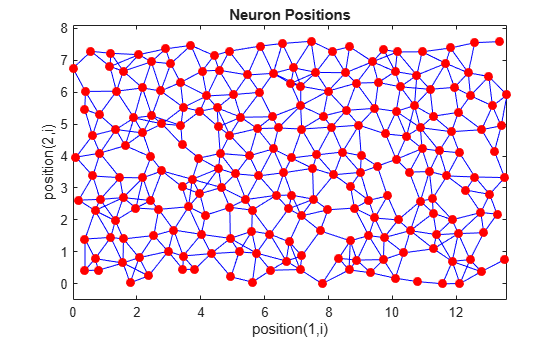Documentation

# randtop

Random layer topology function

## Syntax

```pos = randtop(dimensions) ```

## Description

`randtop` calculates the neuron positions for layers whose neurons are arranged in an `N`-dimensional random pattern.

`pos = randtop(dimensions)` takes one argument:

 `dimensions` Row vector of dimension sizes

and returns an `N`-by-`S` matrix of `N` coordinate vectors, where `N` is the number of dimensions and `S` is the product of `dimensions`.

## Examples

collapse all

This shows how to display a two-dimensional layer with neurons arranged in a random pattern.

```pos = randtop([18 12]); plotsom(pos)```